# Monetizing Vulnerabilities

## From probabilites to dollars and centsRafael Ballestas

In our previous article, we merely scratched the surface of the problem that quantifying risks poses, barely touching on concepts such as calibrated estimation, confidence intervals and specifying the measuring object. Now that (if?) we are convinced that:

• Cybersecurity risk can and should be measured in time-framed probabilities rather than "low", "medium" or "high".

• Estimates should be made not as unrealistic point estimates, but instead as confidence intervals which allow room for uncertainty.

• Even small data can tell us a lot if we use the right tools. Quantitative methods are applicable wherever less precise methods are.

• Experts can make good estimates with the right training.

Let us see how we can turn that into dollars and cents.

Suppose we want to measure the loss incurred by suffering a denial of service attack (the risk). We can decompose the problem into the number of systems affected, the number of hours these are out of service, the revenue streams that rely on these systems, and so a trained expert from your company estimates, with 90% confidence, that the associated loss can fall anywhere between \$2 and \$7 million. This is our calibrated estimate of the impact to the organization.

Now, instead of rating the "likelihood" on a scale from 1 to 5 as is done in the risk "matrices", allow the experts from your security testers to make an estimate in terms of probabilities. Something like "there is a 15% chance that the organization undergoes a denial of service" is what we need to hear here. This particular estimate could be averaged with the ones given by other experts, if need be.

These are all the inputs needed to run a simulation from which we can extract the expected loss associated to a particular risk, the probability of such loss or more, or the overall company-wide loss expected from IT assets, by running a so-called Monte Carlo simulation. In a nutshell, this means that we’re going to simulate the events in a year many times and average the results. A risk happens or not depending on the likelihood given above and the associated costs are taken at random from the above confidence interval.

For a single "simulated year", we just need to generate a random number between 0 and 1. If it is below .15, we say that the event happened. Simple. Now we need to figure out how much we lost. The random number generated above was easy because all numbers between 0 and 1 are equally likely. This is called a uniform distribution. However, in the case of losses, not all numbers are equally likely. We need to generate them according to a different probability distribution which responds to two things: the confidence interval (`CI`) estimated by the expert, and its values should always be positive. The most popular distribution for modeling is the normal distribution, a.k.a. the Gaussian bell: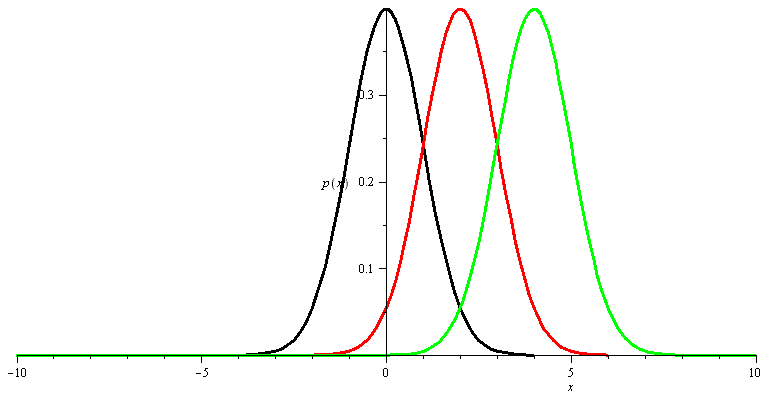Figure 1. Effect of the mean parameter on the normal distribution

However it always allows room for negative values, no matter how much you push the mean parameter towards the positive side, as you see above. For this particular purpose the best fit is the log-normal family of distributions, the distribution of the logarithm of a normally distributed variable.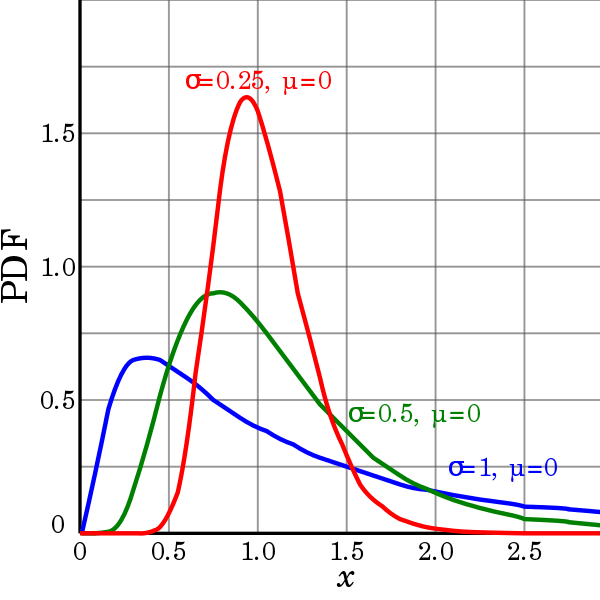Figure 2. Lognormal distributions. Via Wikimedia.

Plus, it has the advantage of being a little skewed towards the lower values, instead of being perfectly symmetric around the mean, which makes lower values more probable and hence is in better correspondence with the reality.

## Get started with Fluid Attacks' Vulnerability Management solution right now

Thus, when the event happens, we can draw a value from the (one and only) lognormal distribution that has the 90% `CI` estimated by the experts, and call that the loss for that risk in that simulated year. We can do the same for other risks, add them all up and so get a global loss due to cybersecurity in that simulated year, thus obtaining a table similar to this one: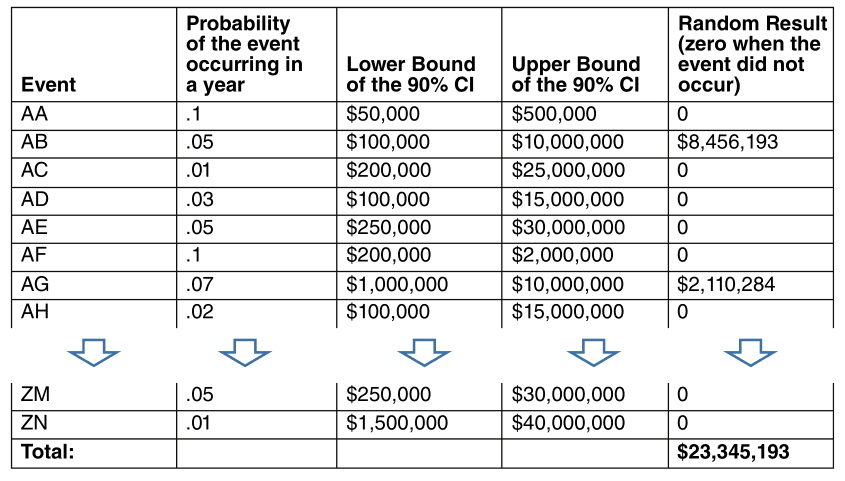Figure 3. Simulated losses for every risk in a "simulated year"

Notice how, given that the probabilities for each risk are small, none larger than 10%, most rows, most of the time, will display a loss of \$0. Now the way to make this into a useful simulation is to run it many times and writing down the results, packed up into bins with which we could make a histogram, or better yet, a curve.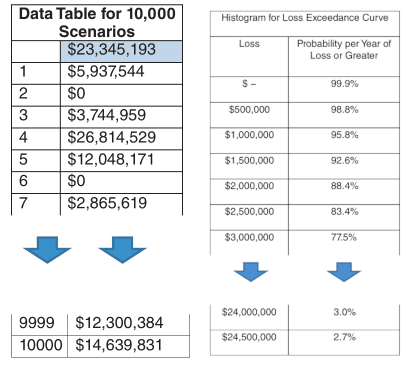Figure 4. Monte Carlo results summary 

From these results, we can build a loss exceedance curve, simply by counting the number of times the simulated results are higher than a threshold, and plotting the results: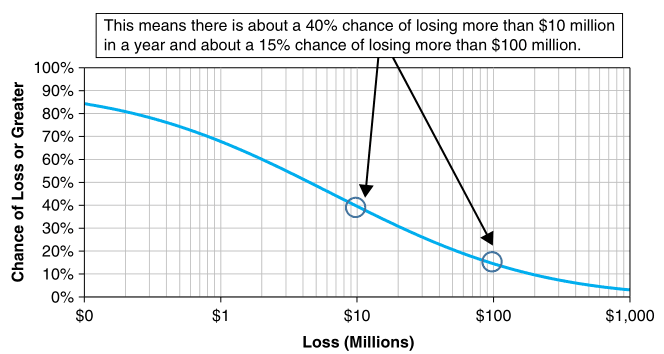Figure 5. Loss Exceedance Curve 

The risk tolerance curve can be obtained from estimation as well, this time by appropriately interviewing an executive expert about the maximum chance with which they would be able to tolerate certain loss thresholds, plotting those risks on a graph, and fitting (interpolating) a curve to these points: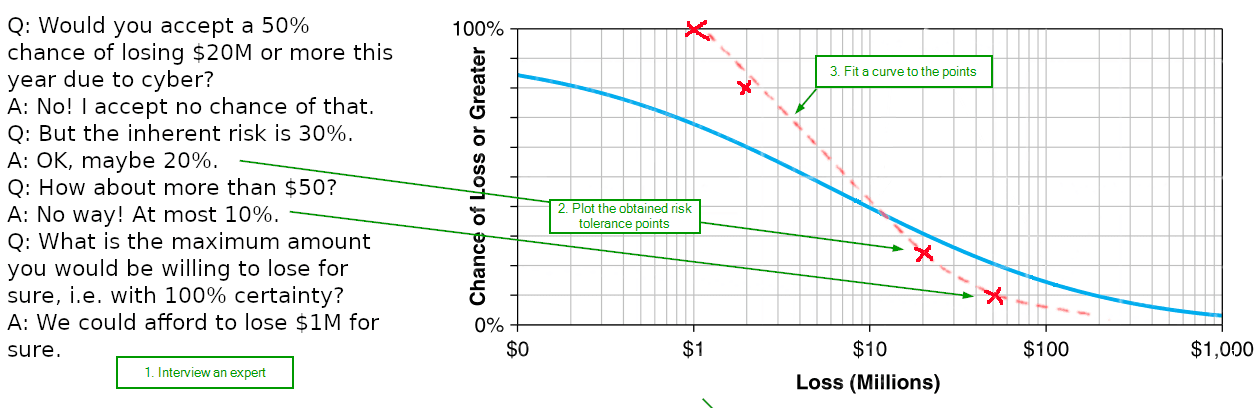Figure 6. Fitting the risk tolerance curve

The residual risk curve corresponds to the probabilities obtained by the same method we used to generate the normal (inherent) risk, only with different occurrence likelihoods estimated by a security expert. So we would just run the Monte Carlo simulation again, only this time with a 1% probability of a denial of service instead of the 15% we used before. Thus we would expect to obtain a curve whose loss probabilities are generally lower than the ones in the inherent risk curve: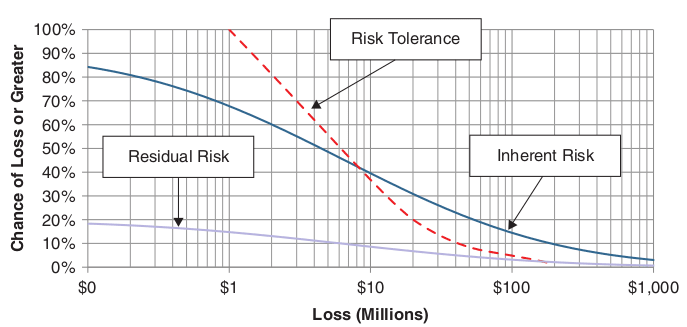Figure 7. All together now 

From these results it is now easier to quantify, compare and finally make a decision regarding which security control assets to invest in. From the Monte Carlo simulations, we could just average the losses to obtain the expected loss. Suppose that is \$10 million. Assume that a certain control, for example, setting up an Intrusion Detection System, makes the probability of the denial of service lower to 8%, and the probability of some of other events go down as well. If we run the simulation again, we will obtain a different, most likely lower, expected loss. Call it \$8M, and suppose the `IDS` costs \$0.5M. In that case we might say that the return on the control is given by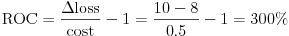Figure 8. Return on control

Clearly, an investment that reduces the expected loss by four times its cost is clearly worth it. Try to compare that cost to having 2 red-colored risks and 3 yellow ones.

To recap, this loss exceedance curve is obtained by running several simulations which, in turn, feed from two expert estimates: the estimated cost of a security event, reported as a confidence interval, and the probability of an event occurring, given the context in which such a vulnerability would happen. The first can be easily estimated internally, but the second can only be determined from a thorough security audit and penetration testing. From the above numbers, which are of course made up, but not so out of this world, you can make a more informed decision regarding the investments your organization is willing to make regarding both offensive and defensive security. With the above tool, you have the gist to make these simulations yourself.

## References

1. D. Hubbard, R. Seiersen (2016). How to measure anything in cibersecurity risk. Wiley.

2. S. Latchman (2010). Quantifying the Risk of Natural Catastrophes. Understanding Uncertainty.

3. quantmleap (2010). Project Risk Management and the application of Monte Carlo Simulation.

Share

Tags:

## Recommended blog posts

You might be interested in the following related posts.Tips for choosing a vulnerability management solutionDefinition, implementation, importance and alternativesKeep tabs on this proposal from the Biden-Harris AdminVulnerability scanning and pentesting for a safer webDefinitions, classifications and pros and consIs your security testing covering the right risks?How this process works and what benefits come with itGet an overview of vulnerability assessment

## Start your 21-day free trial

Discover the benefits of our Continuous Hacking solution, which hundreds of organizations are already enjoying.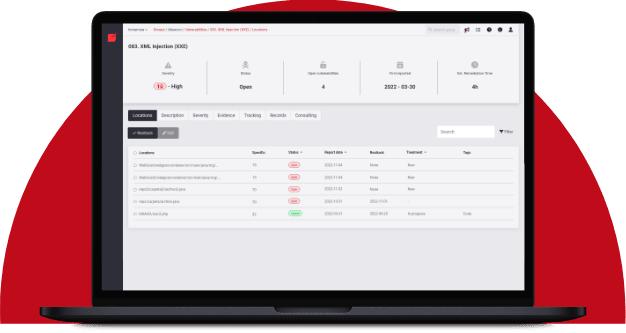Hacking software for over 20 years

Fluid Attacks tests applications and other systems, covering all software development stages. Our team assists clients in quickly identifying and managing vulnerabilities to reduce the risk of incidents and deploy secure technology.